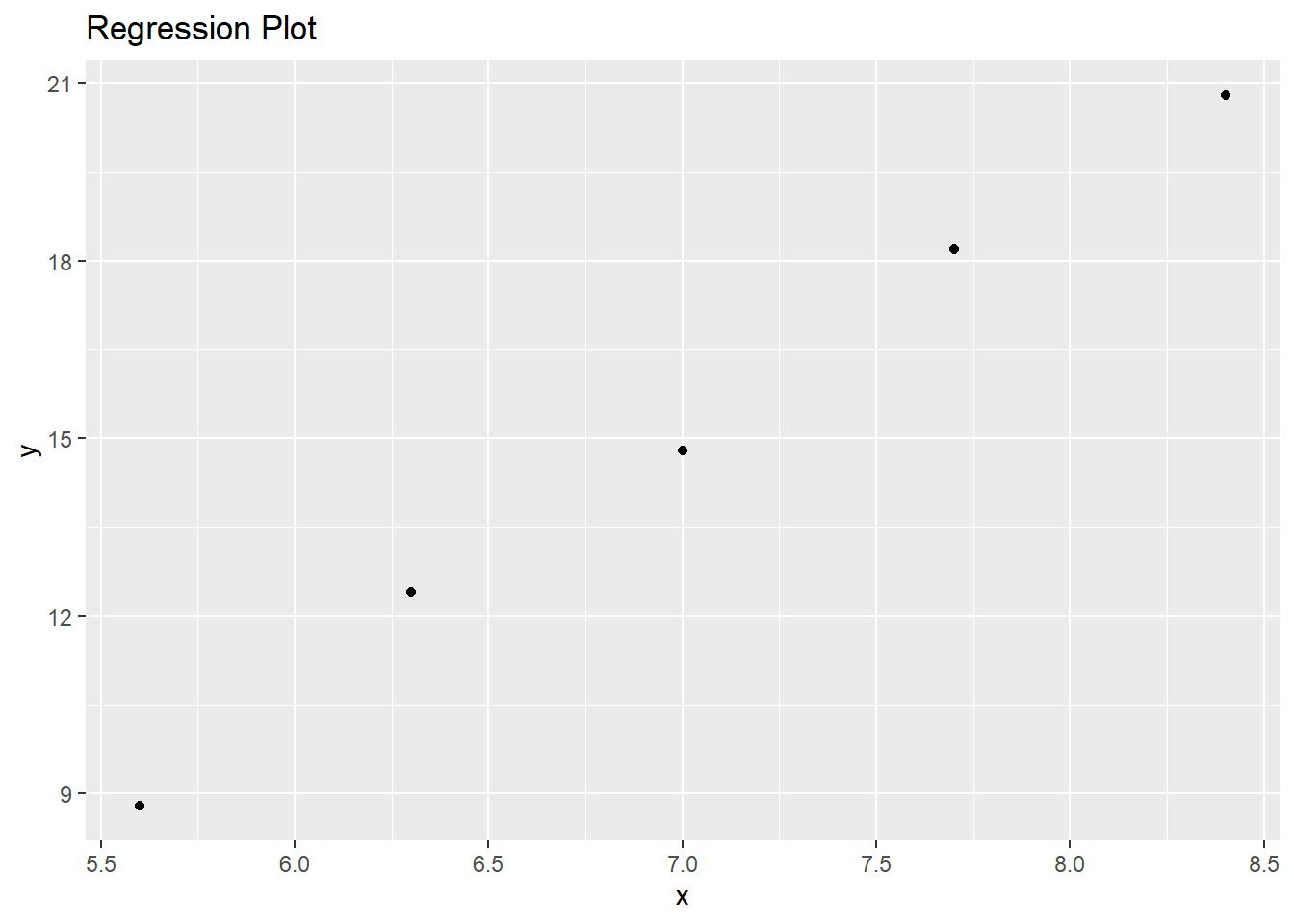# Calculus Assignment 15

library(ggplot2)

## Question 1

Find the equation of the regression line for the given points. Round any final values to the nearest hundredth, if necessary. (5.6, 8.8), (6.3, 12.4), (7, 14.8), (7.7, 18.2), (8.4, 20.8)

First, we create lists containing the x and y values of the given points.

x <- c(5.6, 6.3, 7, 7.7, 8.4)
y <- c(8.8, 12.4, 14.8, 18.2, 20.8)
reg <- cbind(x, y)
reg
##        x    y
## [1,] 5.6  8.8
## [2,] 6.3 12.4
## [3,] 7.0 14.8
## [4,] 7.7 18.2
## [5,] 8.4 20.8

We can visualize these points in a scatter plot.

ggplot(reg, aes(x, y)) + geom_point() + ggtitle("Regression Plot")The regression line for these points is:

$y = 4.257x - 14.8$

reg_line <- lm(y~x)
print(reg_line)
##
## Call:
## lm(formula = y ~ x)
##
## Coefficients:
## (Intercept)            x
##     -14.800        4.257

## Question 2

Find all local maxima, local minima, and saddle points for the function given below. Write your answer(s) in the form (x, y, z). Separate multiple points with a comma.

$f(x,y) = 24x - 6xy^2 - 8y^3$

#### Find the partial derivatives:

$f_x = 24 - 6y^2$ $f_y = 12xy - 24y^2$

#### Find the critical points:

Critical points occur when both partial derivatives are equal to 0.

$24 - 6y^2 = 0$ $y = -2, 2$

Plug in y = -2 and y = 2 into the following equation and find x. $12xy - 24y^2 = 0$

y = -2 $-24x - 96 = 0 (x = -4)$

y = 2 $24y - 96 = 0 (x = 4)$

#### Values at critical points

f1 <- function(x,y){
return(24*x - 6*x*y^2 - 8*y^3)
}
f1(-4, -2)
##  64
f1(4, 2)
##  -64

The critical points are (-4, -2, 64) and (4, 2, -64)

#### Find second order partial derivatives:

$f_{xx} = 0$ $f_{yy} = 12x - 48y$ $f_{xy} = 12y$

#### Find D

D at (-4, -2, 64) $D = f_{xx}(-4, -2)f_{yy}(-4, -2) - f_{xy}^2(-4, -2) = ((48 * 0) - 12 * (-2))^2 = 576$

D at (4, 2, -64) $D = f_{xx}(4, 2)f_{yy}(4, 2) - f_{xy}^2(4, 2) = ((-48 * 0) - 12 * 2)^2 = 576$

Since D > 0 for both critical points, there are no saddle points.

(-4, -2, 64) is a minimum point because fyy > 0. (4, 2, -64) is a maximum point because fyy < 0.

## Question 3

A grocery store sells two brands of a product, the “house” brand and a “name” brand. The manager estimates that if she sells the “house” brand for x dollars and the “name” brand for y dollars, she will be able to sell 81  21x + 17y units of the “house” brand and 40 + 11x  23y units of the “name” brand.

Step 1. Find the revenue function R (x, y).

R(x,y) = house units * house price + name units * name price $R(x,y) = x(81 - 21x + 17y) + y(40 + 11x - 23y) = 81x - 21x^2 + 28xy + 40y - 23y^2$

Step 2. What is the revenue if she sells the “house” brand for $2.30 and the “name” brand for$4.10?

rev_fun <- function(x, y){
return(81*x - 21*x^2 + 28*x*y + 40*y - 23*y^2)
}

rev_fun(2.30, 4.10)
##  116.62

The revenue would be \$116.62.

## Question 4

A company has a plant in Los Angeles and a plant in Denver. The firm is committed to produce a total of 96 units of a product each week. The total weekly cost is given by $C(x, y) = \frac{1}{6}x^2 + \frac{1}{6}y^2 + 7x + 25y + 700$ , where x is the number of units produced in Los Angeles and y is the number of units produced in Denver. How many units should be produced in each plant to minimize the total weekly cost?

x + y = 96

$C_x = \frac{1}{3}x + 7$ $C_y = \frac{1}{3}y + 25$

$\frac{1}{3}x + 7 = \frac{1}{3}y + 25$

$x = y + 54 | x + y = 96$

x = 75 y = 21

## Question 5

Evaluate the double integral on the given region.

$\int \int_R e^{8x+3y}dA$ Region A - x: [2,4] y: [2,4]

$\int_{2}^{4} \int_{2}^{4} (e^{8x+3y})dx dy$ $\int_{2}^{4} \int_{2}^{4} (e^{8x+3y})dx dy$
$\frac{1}{8}\int_{2}^{4} e^{32+3y} - e^{16+3y}dy$
$\frac{1}{8}[\frac{1}{3}e^{32+3y} - \frac{1}{3}e^{16+3y}]^4_2$
$\frac{1}{8}[(\frac{1}{3}e^{32+12} - \frac{1}{3}e^{16+12}) - (\frac{1}{3}e^{32+6} - \frac{1}{3}e^{16+6})]$
$\frac{1}{24}(e^{44} - e^{28} - e^{38} + e^{22})$